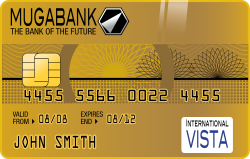# The Codabar Check

##### Age 11 to 14

Published 2000 Revised 2011Every credit card is uniquely identified by a 16-digit number. The first 15 digits are assigned by the bank issuing the card. The last digit (called the check digit) is determined by a formula which enables a computer to check that the number has been correctly entered when the card is used in a transaction.

All the major credit card companies use a system called Codabar to assign the check digit. This is how it works.

1. Suppose that the first 15 digits are

$3141 5926 5358 979$

2. Add the digits in the odd positions and double the total.

$(3+4+5+2+5+5+9+9)\times 2=84$

3. Add the digits in the even positions.

$1+1+9+6+3+8+7=35$

4. Count the number of digits in the odd positions that are greater than $4$. The numbers in this example are $5$, $5$, $5$, $9$ and $9$, that is altogether $5$ numbers.

5. Now add together the three numbers obtained in this way to get $84+35+5=124$.

6. The check digit is the number needed to bring this total up to the next multiple of $10$. What should this be in our example? Yes, the required check digit is $6$.

The Codabar system is one of the most efficient error detection methods. It picks up 100% of all single digit errors, and most other common errors such as switching two adjacent digits.

Have a look at some credit cards for yourself and you will find that the last digit is given by the formula described here. Test it to see if it picks up errors likely to be made in transcribing the number.